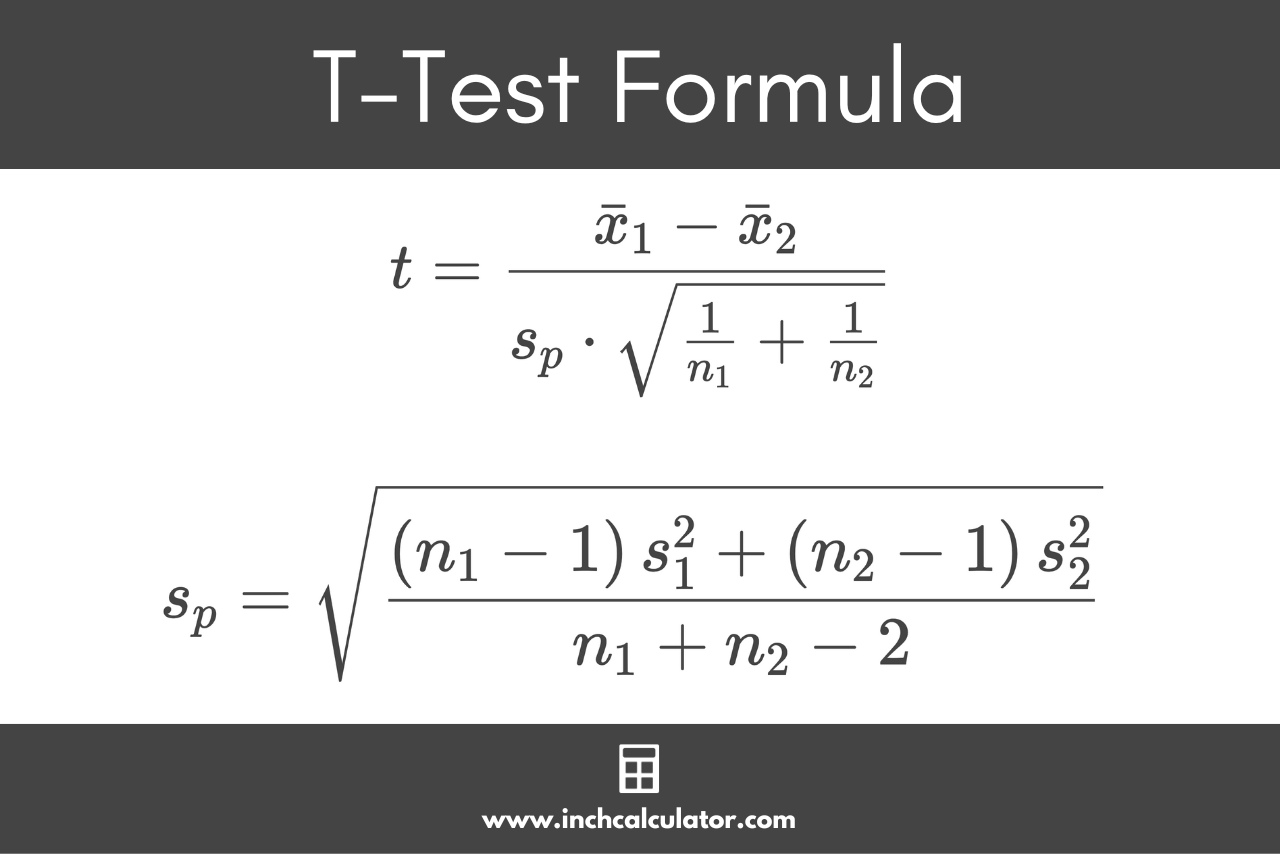# T-Test Calculator

Compare the means of two samples using a single-sample or two-sample t-test below.

Sample:
Sample One:
Sample Two:

## Results:

Learn how we calculated this below

## How to do a T-Test

A t-test calculates how significant the difference between the means of two groups are. The results let you know if those differences could have occurred by chance, or rather, whether the difference is statistically significant.

A t-test uses the test statistic, sometimes called a t-value or t-score, the t-distribution values, and the degrees of freedom to calculate the statistical significance of the difference.

Since a t-test is a parametric test, it will only provide useful results if the data is normally distributed and each data point is independent.

### Types of T-Tests

The first part of doing a t-test is determining which type of t-test you need to do.

There are three different types of t-tests:

• one-sample t-test: used to compare the mean of a sample to the known mean of a population
• two-sample t-test: used to compare the mean of two different independent samples
• paired t-test: used to compare the mean of two different samples after an intervention or change

### How to Calculate T Using a One-Sample T-Test

A one-sample t-test, or single-sample test, is use to the compare a sample mean to a population mean when the null hypothesis is that the sample mean is equal to the population mean.

#### One-Sample T-Test Formula

To calculate the t value using a one-sample t-test, use the following formula:

t=\frac{\bar{x}-\mu}{s/\sqrt{n}}

Where:
= sample mean
μ = population mean
s = sample standard deviation
n = sample size

Thus, the test statistic t is equal to the difference between the sample mean and the population mean μ, divided by the standard error s / √n.

### How to Calculate T Using a Student’s T-Test

A Student’s t-test is used for test statistics that follow a Student’s t-distribution under the null hypothesis that two populations have equal means. The Student’s t-test assumes that the variances of two populations are equal.

This is a type of two-sample test used to compare two sample means, where a large t-value suggests that the samples are very different and a small t-value suggests that they are similar.

#### Student’s T-Test Formula

The formula for a Student’s t-test is:

t=\frac{\bar{x}_{1}-\bar{x}_{2}}{s_{p} \cdot \sqrt{\frac{1}{n_{1}}+\frac{1}{n_{2}}}}

Given the formula to calculate the pooled standard deviation sp:

s_{p}=\sqrt{\frac{\left ( n_{1}-1 \right ) s_{1}^{2}+\left ( n_{2}-1 \right ) s_{2}^{2}}{n_{1}+n_{2}-2}}

Where:
1 = first sample mean
2 = second sample mean
n1 = first sample size
n2 = second sample size
s1 = first sample standard deviation
s2 = second sample standard deviation
n1 + n2 – 2 = degrees of freedom ν

In a Student’s t-test, the test statistic t is equal to the difference between sample means 1 and 2, divided by the pooled standard deviation sp times the square root of 1 divided by the first sample size n1 plus 1 divided by the second sample size n2.

The pooled standard deviation sp is equal to the first sample size n1 minus 1 times the first sample standard deviation s1 plus the second sample size n2 minus 1 times the second sample standard deviation s2, divided by the degrees of freedom, in this case the sum of the sample sizes minus two.

This formula can be broken down into a few simple steps.

#### Step One: Calculate the Degrees of Freedom

\nu=n_{1}+n_{2}-2

#### Step Two: Calculate the Pooled Standard Deviation

s_{p}=\sqrt{\frac{\left ( n_{1}-1 \right ) s_{1}^{2}+\left ( n_{2}-1 \right ) s_{2}^{2}}{\nu}}

#### Step Three: Calculate the Test Statistic

t=\frac{\bar{x}_{1}-\bar{x}_{2}}{s_{p} \cdot \sqrt{\frac{1}{n_{1}}+\frac{1}{n_{2}}}}### How to Calculate T Using Welch’s T-Test

Recall that the Student’s t-test assumes that the variances of two populations are equal. But, what if you need to perform a t-test given two populations with different variances?

In this case, you can use Welch’s t-test, which is sometimes also called an unequal variances t-test. Like before the null hypothesis with this test is that two populations have equal means.

#### Welch’s T-Test Formula

The formula for Welch’s t-test is:

t=\frac{\bar{x}_{1}-\bar{x}_{2}}{\sqrt{\frac{s_{1}^{2}}{n_{1}}+\frac{s_{2}^{2}}{n_{2}}}}

#### Degrees of Freedom Formula

To find the degrees of freedom when using Welch’s t-test, use the Satterthwaite formula:

\nu=\frac{\left ( s_{1}^{2}/n_{1}+s_{2}^{2}/n_{2} \right )^{2}}{\frac{ \left ( s_{1}^{2}/n_{1} \right )^{2} }{n_{1}-1}+\frac{ \left ( s_{2}^{2}/n_{2} \right )^{2} }{n_{2}-1}}

### Find the P-Value

The next step is to find the p-value for the test statistic. A p-value is used to determine whether to reject the null hypothesis.

Use a t-table and locate the degrees of freedom in the left-most column. Then, locate the desired p-value in the heading row, 0.05 is most commonly used for a 95% confidence level.

Then, find the intersection of the row and column to find the critical value.

If the calculated t-value is larger than the critical value, then you can reject the null hypothesis. If it is less than the critical value, then you fail to reject the null hypothesis.